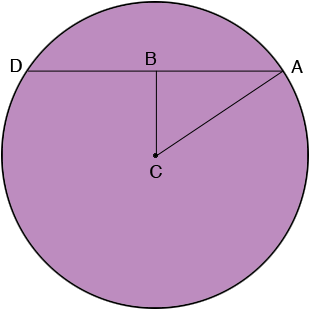SEARCH HOMEMath Central Quandaries & QueriesQuestion from Dakota, a student: If the diameter of a circle is 48 cm, find the shortest distance from a chord of length 34 cm to the center of the circleHi Dakota,

In my diagram the chord is AD, C is the center of the circle and B is the midpoint of the chord.Angle ABC is a right angle. an you see why? The length of CB is the shortest distance from C to the chord. Can you see why?

What does Pythagoras theorem tell you?

PennyMath Central is supported by the University of Regina and the Imperial Oil Foundation.